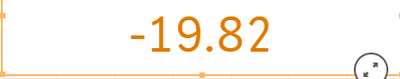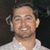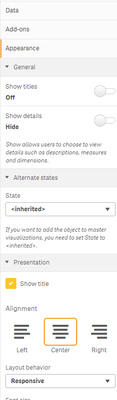# New to Qlik Sense

If you’re new to Qlik Sense, start with this Discussion Board and get up-to-speed quickly.

Announcements
Our next Qlik Insider session will cover new key capabilities. Join us August 11th REGISTER TODAY
cancel
Showing results for
Did you mean:Contributor III

## Qlik Sense - Remove any decimals when formatting

Hi Qlikers,

I have a challenge on a minus number percentage without any decimals

Currently whats showing,What want to see is =    -19%

The measure I used to get the above is

(Round(Num(Count({<FYear_Num={\$(=Max(FYear_Num))}>} Incident_Number),)/(date(today(),'DD/MM/YYYY')-'01/10/2019'))/
(Num(Count({<FYear_Num={\$(=Max(FYear_Num)-1)}>} Incident_Number),)/365)-1)* 100

AND

(Ceil(Num(Count({<FYear_Num={\$(=Max(FYear_Num))}>} Incident_Number),)/(date(today(),'DD/MM/YYYY')-'01/10/2019'))/
(Num(Count({<FYear_Num={\$(=Max(FYear_Num)-1)}>} Incident_Number),)/365)-1)* 100

What I have tried so far but unsuccessful using example from community.

//((Sum(Sales)/Sum(Products)),'#0%').
//num(sum(Value)/sum(total Value), '#,##0%')
//=Value&'%'
//round(Count({< [Escalated Case Count] = {1}>} DISTINCT CustomID) / SUM ([Open Count]))
//num(AVG([Total CTR]), '#.##%')

Below is where I used above community given examples

(Round(Num(Count({<FYear_Num={\$(=Max(FYear_Num))}>} Incident_Number),'Unsucessful')/(date(today(),'DD/MM/YYYY')-'01/10/2019'))/
(Num(Count({<FYear_Num={\$(=Max(FYear_Num)-1)}>} Incident_Number),'Unsucessful')/365)-1)* 100

Anyone can help would be really appreciated. Thanks

Labels (4)

• ### SaaS

1 Solution

Accepted SolutionsCreator III

In the measure you should just be able to turn number formatting off of auto, turn on Number, and choose no decimal places.

5 RepliesCreator III

Are you trying to do this in a KPI or table or what chart?Creator III

In the measure you should just be able to turn number formatting off of auto, turn on Number, and choose no decimal places.Contributor III
Author

Hi Steven I was trying to do a KPIContributor III
Author

That is my question to be honest. I am Not sure how I can do that.

Below is what I see,Contributor III
Author

I got it now. ThanksTags
Community Browser